# Friction Forces (Part - 2) Notes - Civil Engineering (CE)

## Civil Engineering (CE): Friction Forces (Part - 2) Notes - Civil Engineering (CE)

The document Friction Forces (Part - 2) Notes - Civil Engineering (CE) is a part of Civil Engineering (CE) category.
All you need of Civil Engineering (CE) at this link: Civil Engineering (CE)

2.5.3 Experimental values for friction coefficient

The table below (taken from `Engineering Materials’ by Ashby and Jones, Pergammon, 1980) lists rough values for friction coefficients for various material pairs.

 Material Approx friction coefficient Clean metals in air 0.8-2 Clean metals in wet air 0.5-1.5 Steel on soft metal (lead, bronze, etc) 0.1-0.5 Steel on ceramics (sapphire, diamond, ice) 0.1-0.5 Ceramics on ceramics (eg carbides on carbides) 0.05-0.5 Polymers on polymers 0.05-1.0 Metals and ceramics on polymers (PE, PTFE, PVC) 0.04-0.5 Boundary lubricated metals (thin layer of grease) 0.05-0.2 High temperature lubricants (eg graphite) 0.05-0.2 Hydrodynamically lubricated surfaces (full oil film) 0.0001-0.0005

These are rough guides only – friction coefficients for a given material can by highly variable. For example, Lim and Ashby (Cambridge University Internal Report CUED/C-mat./TR.123 January 1986) have catalogued a large number of experimental measurements of friction coefficient for steel on steel, and present the data graphically as shown below. You can see that friction coefficient for steel on steel varies anywhere between 0.0001 to 3.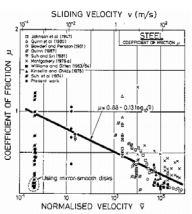Friction coefficient can even vary significantly during a measurement. For example, the picture below (from Lim and Ashby, Acta Met 37 3 (1989) p 767) shows the time variation of friction coefficient during a pin-on-disk experiment.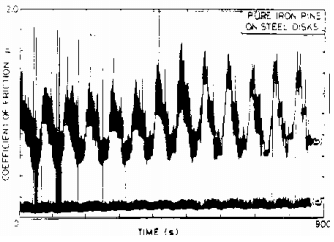2.5.4 Static and kinetic friction

Many introductory statics textbooks define two different friction coefficients. One value, known as the coefficient of static friction and denoted by μs , is used to model static friction in the equation giving the condition necessary to initiate slip at a contact

|T| < μsN

A second value, known as the coefficient of kinetic friction, and denoted by μk , is used in the equation for the force required to maintain steady sliding between two surfaces

T = ±μN

I don’t like to do this (I’m such a rebel). It is true that for some materials the static friction force can be a bit higher than the kinetic friction force, but this behavior is by no means universal, and in any case the difference between μk and μs is very small (of the order of 0.05). We’ve already seen that μ can vary far more than this for a given material pair, so it doesn’t make much sense to quibble about such a small difference.

The real reason to distinguish between static and kinetic friction coefficient is to provide a simple explanation for slip-stick oscillations between two contacting surfaces. Slip-stick oscillations often occur when we try to do the simple friction experiment shown below.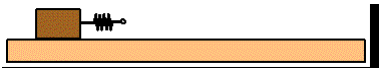If the end of the spring is moved steadily to the right, the block sticks for a while until the force in the spring gets large enough to overcome friction. At this point, the block jumps to the right and then sticks again, instead of smoothly following the spring. If μ were constant, then this behavior would be impossible. By using μs > μk , we can explain it. But if we’re not trying to model slip-stick oscillations, it’s much more sensible to work with just one value of μ .

In any case, there’s a much better way to model slip-stick oscillations, by making μ depend on the velocity of sliding. Most sophisticated models of slip-stick oscillations (e.g. models of earthquakes at faults) do this.

12.6 The microscopic origin of friction forces

Friction is weird. In particular, we need to explain
(i) why friction forces are independent of the contact area
(ii) why friction forces are proportional to the normal force.

Coulomb grappled with these problems and came up with an incorrect explanation. A truly satisfactory explanation for these observations was only found 20 years or so ago.

To understand friction, we must take a close look at the nature of surfaces. Coulomb/Amonton friction laws are due to two properties of surfaces:

(1) All surfaces are rough;
(2) All surfaces are covered with a thin film of oxide, an adsorbed layer of water, or an organic film.

Surface roughness can be controlled to some extent – a cast surface is usually very rough; if the surface is machined the roughness is somewhat less; roughness can be reduced further by grinding, lapping or polishing the surfaces. But you can’t get rid of it altogether. Many surfaces can be thought of as having a fractal geometry. This means that the roughness is statistically self-similar with length scale – as you zoom in on the surface, it always looks (statistically) the same (more precisely the surfaces are self-affine. When you zoom in, it looks like the surface got stretched vertically – surfaces are rougher at short wavelengths than at long ones).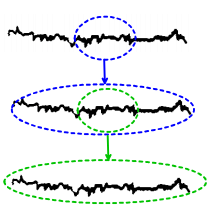Of course no surface can be truly fractal: roughness can’t be smaller than the size of an atom and can’t be larger than the component; but most surfaces look fractal over quite a large range of lengths. Various statistical measures are used to quantify surface roughness, but a discussion of these parameters is beyond the scope of this course.

Now, visualize what the contact between two rough surfaces looks like. The surfaces will only touch at high spots (these are known in the trade as `asperities’) on the two surfaces. Experiments suggest that there are huge numbers of these contacts (nobody has really been able to determine with certainty how many there actually are). The asperity tips are squashed flat where they contact, so that there is a finite total area of contact between the two surfaces. However, the true contact area (at asperity tips) is much smaller than the nominal contact area.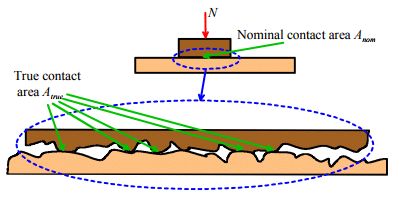The true contact area can be estimated by measuring the surface roughness, and then calculating how the surfaces deform when brought into contact. At present there is some uncertainty as to how this should be done – this is arguably the most important unsolved problem in the field. The best estimates we have today all agree that:

The true area of contact between two rough surfaces is proportional to the normal force pressing them together

Atrue = CN

At present, there is no way to measure or calculate the contact C accurately.

This is true for all materials (except for rubbers, which are so compliant that the true contact area is close to the nominal contact area), and is just a consequence of the statistical properties of surface roughness. The reason that the true contact area increases in proportion to the load is that as the surfaces are pushed into contact, the number of asperity contacts increases, but the average size of the contacts remains the same, because of the fractal self-similarity of the two surfaces.

Finally, to understand the cause of the Coulomb/Amonton friction law, we need to visualize what happens when two rough surfaces slide against each other.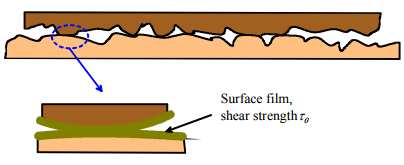Each asperity tip is covered with a thin layer of oxide, adsorbed water, or grease. It’s possible to remove this film in a lab experiment – in which case friction behavior changes dramatically and no longer follows Coulomb/Amonton law – but for real engineering surfaces it’s always present.

The film usually has a low mechanical strength. It will start to deform, and so allow the two asperities to slide past each other, when the tangential force per unit area acting on the film reaches the shear strength of the film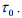The tangential friction force due to shearing the film on the surface of all the contacting asperities is therefore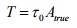Combining this with the earlier result for the true contact area gives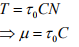Thus, the friction force is proportional to the normal force. This simple argument also explains why friction force is independent of contact area; why it is so sensitive to surface films, and why it can be influenced (albeit only slightly) by surface roughness.

The document Friction Forces (Part - 2) Notes - Civil Engineering (CE) is a part of Civil Engineering (CE) category.
All you need of Civil Engineering (CE) at this link: Civil Engineering (CE)Use Code STAYHOME200 and get INR 200 additional OFF

Track your progress, build streaks, highlight & save important lessons and more!

,

,

,

,

,

,

,

,

,

,

,

,

,

,

,

,

,

,

,

,

,

;